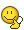BrainDen.com - Brain Teasers
• 0## Questionan algorithm for determining if a divides b would be the following.

if a is odd:

if b is evenly divisable by 2, divide by 2.

else subtract a.

repeat untill you get a value between 0 and a. this is the modulus.

is there a similar such algorithm if a is even?

(technically the above one does work, but it's rather slow if b is odd, find me a fast one.)

## Recommended Posts

• 0edit: the above algorithm does NOT give the modulus, sorry. it does however work as a divisability detector.

##### Share on other sites

• 0

#1 The best algorithm is to divide b by a and check if reminder is zero or not.#2 If division and multiplication is not allowed, then keep subtracting a from b while b<=a and check if the end value of b is 0.

#3 If only division by 2 is allowed, then method #2 can be improved by dividing both a and b by 2 in a loop while both a and b are even (prior to applying #2). If the above reduction terminates with even a and odd b, then there is an immediate answer "NO". If the above reduction terminates with odd a and even b, then keep dividing b by 2 until it is odd. Only with both a and b odd proceed to #2. Ah, and do not forget to check for special cases (a=0, a=1, a=2) to begin with.Edited by witzar
##### Share on other sites

• 0

#4 If addition (or multiplication by 2) is allowed, then #2 (from my post #3) can by significantly improved (in terms of computational complexity of the algorithm).

##### Share on other sites

• 0if a is even and b is odd it is not divisible## Join the conversation

You can post now and register later. If you have an account, sign in now to post with your account.×   Pasted as rich text.   Paste as plain text instead

Only 75 emoji are allowed.

×   Your previous content has been restored.   Clear editor

×   You cannot paste images directly. Upload or insert images from URL.